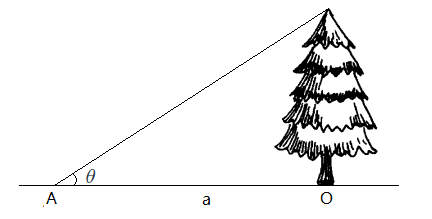安卓手机扫描二维码安装AppA. 取 ﻿$\theta=\dfrac{\pi}{3}$﻿ , 即 ﻿$a=\dfrac{\sqrt{3}}{3}h$﻿

B. 取 ﻿$\theta=\dfrac{\pi}{4}$﻿ , 即 ﻿$a=h$﻿

C. 取 ﻿$\theta=\dfrac{\pi}{6}$﻿ , 即 ﻿$a=\sqrt{3}h$﻿苹果手机扫描二维码安装App